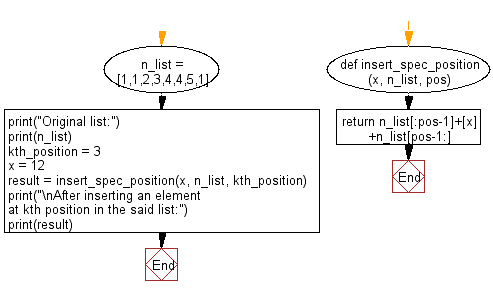﻿ Python: Insert an element at a specified position into a given list - w3resource

# Python: Insert an element at a specified position into a given list

## Python List: Exercise - 80 with Solution

Write a Python program to insert an element at a specified position into a given list.

Sample Solution:

Python Code:

``````def insert_spec_position(x, n_list, pos):
return n_list[:pos-1]+[x]+n_list[pos-1:]
n_list = [1,1,2,3,4,4,5,1]
print("Original list:")
print(n_list)
kth_position = 3
x = 12
result = insert_spec_position(x, n_list, kth_position)
print("\nAfter inserting an element at kth position in the said list:")
print(result)
```
```

Sample Output:

```Original list:
[1, 1, 2, 3, 4, 4, 5, 1]

After inserting an element at kth position in the said list:
[1, 1, 12, 2, 3, 4, 4, 5, 1]
```

Flowchart:## Visualize Python code execution:

The following tool visualize what the computer is doing step-by-step as it executes the said program:

Python Code Editor:

Have another way to solve this solution? Contribute your code (and comments) through Disqus.

What is the difficulty level of this exercise?

Test your Programming skills with w3resource's quiz.

﻿

## Python: Tips of the Day

Decapitalizes the first letter of a string:

Example:

```def tips_decapitalize(s, upper_rest=False):
return s[:1].lower() + (s[1:].upper() if upper_rest else s[1:])
print(tips_decapitalize('PythonTips'))
print(tips_decapitalize('PythonTips', True))
```

Output:

```pythonTips
pYTHONTIPS
```

We are closing our Disqus commenting system for some maintenanace issues. You may write to us at reach[at]yahoo[dot]com or visit us at Facebook